StatLect

# Log-normal distribution

A random variable is said to have a log-normal distribution if its natural logarithm has a normal distribution. In other words, the exponential of a normal random variable has a log-normal distribution.## Definition

Log-normal random variables are characterized as follows.

Definition Letbe a continuous random variable. Let its support be the set of strictly positive real numbers:We say thathas a log-normal distribution with parametersandif its probability density function is## Relation to the normal distribution

The relation to the normal distribution is stated in the following proposition.

Proposition Letbe a normal random variable with meanand variance. Then the variablehas a log-normal distribution with parametersand.

Proof

Ifhas a normal distribution, then its probability density function isThe functionis strictly increasing, so we can use the formula for the density of a strictly increasing functionIn particular, we haveso that## Expected value

The expected value of a log-normal random variableisProof

It can be derived as follows: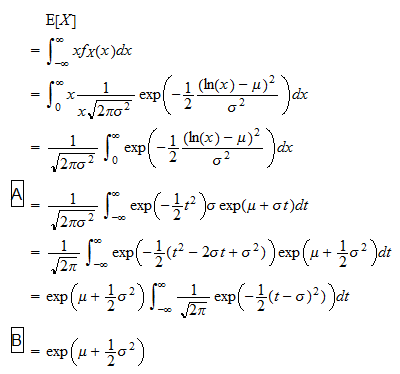where: in stepwe have made the change of variableand in stepwe have used the fact thatis the density function of a normal random variable with meanand unit variance, and as a consequence, its integral is equal to 1.

## Variance

The variance of a log-normal random variableisProof

Let us first derive the second moment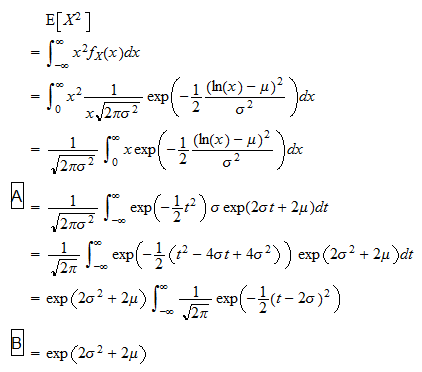where: in stepwe have made the change of variable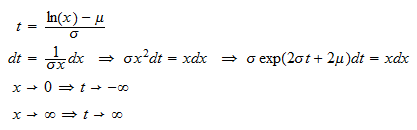and in stepwe have used the fact thatis the density function of a normal random variable with meanand unit variance, and as a consequence, its integral is equal to 1. We can now use the variance formula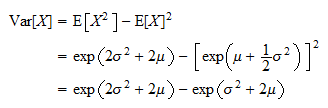## Higher moments

The-th moment of a log-normal random variableisProof

It can be derived as follows:where: in stepwe have made the change of variable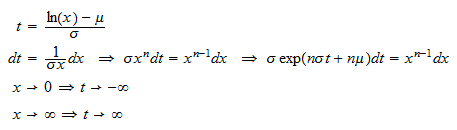and in stepwe have used the fact thatis the density function of a normal random variable with meanand unit variance, and as a consequence, its integral is equal to 1.

## Moment generating function

The log-normal distribution does not possess the moment generating function.

## Characteristic function

A closed formula for the characteristic function of a log-normal random variable is not known.

## Distribution function

The distribution functionof a log-normal random variablecan be expressed aswhereis the distribution function of a standard normal random variable.

Proof

We have proved above that a log-normal variablecan be written aswherehas a normal distribution with meanand variance. In turn,can be written aswhereis a standard normal random variable. As a consequence,